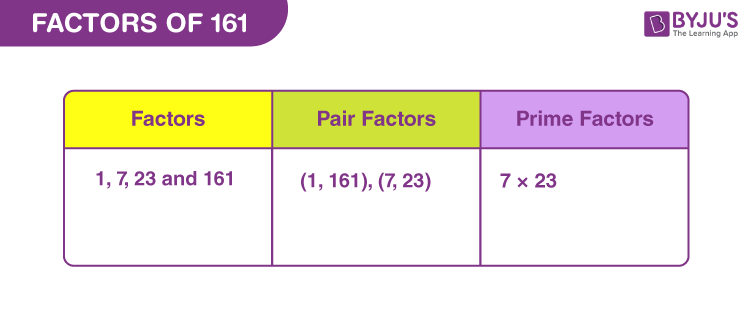# Factors of 161

The factors of 161 are the numbers that are multiplied in pairs resulting in an original number 161. In other words, the numbers that divide 161 exactly without leaving a remainder value are the factors of 161. The factors and the pair factors of 161 can be positive or negative, but they cannot be a decimal or fractional number. For example, the pair factor of 161 is expressed as (1, 161) or (-1, -161). The multiplication of pair of negative numbers, such as multiplying -1 and -161, will result in the original number.Here, we are going to learn what are the factors of 161, the positive and negative pair factors of 161, prime factorization of 161 and solved examples.

## What are the Factors of 161?

In Mathematics, the factors of a number are the numbers that divide the given number exactly and leaves a remainder 0. Thus, the factors of 161 are the numbers that divide 161 completely without leaving any remainder. As 161 is a composite number, it has more than two factors. Therefore, the factors of 161 are 1, 7, 23 and 161. Similarly, the negative factors of 161 are -1, -7, -23 and -161.

 Factors of 161: 1, 7, 23 and 161. Prime Factorization of 161: 7 × 23.

## Pair Factors of 161

The numbers that are multiplied together in pairs resulting in the number 161 are the pair factors of 161. The following are the positive and negative pair factors of 161.

Positive Pair Factors of 161:

 Positive Factors of 161 Positive Pair Factors of 161 1 × 161 (1, 161) 7 × 23 (7, 23)

Therefore, the positive pair factors of 161 are (1, 161) and (7, 23).

Negative Pair Factors of 161:

 Negative Factors of 161 Negative Pair Factors of 161 -1 × -161 (-1, -161) -7 × -23 (-7, -23)

Therefore, the negative pair factors of 161 are (-1, -161) and (-7, -23).

## Factors of 161 by Division Method

The factors of 161 are found using the division method. In the division method, divide the number 161 by a different integer. If the integer divides 161 exactly and leaves a remainder 0, then the integer is a factor of 161. Now, let us start dividing 161 by 1 and then continue with the different integers.

• 161/1 = 161 (Factor is 1 and Remainder is 0)
• 161/7 = 23 (Factor is 7 and Remainder is 0)
• 161/23 = 7 (Factor is 23 and Remainder is 0)
• 161/161 = 1 (Factor is 161 and Remainder is 0)

If we divide 161 by any numbers other than 1, 7, 23 and 161, it leaves a remainder of some value. Hence, the factors of 161 are 1, 7, 23 and 161.

## Prime Factorization of 161

The method of writing the number 161 as the product of its prime factors is called the prime factorization of 161. Go through the following procedure to find the prime factors of 161 using the prime factorization method.

Consider a pair factor of 161, say (1, 161)

We know that the number 161 is a composite number, and it can be split further into its prime factors. Thus, the number 161 is written as the product of 7 and 23, where 7 and 23 are the prime numbers.

Therefore, the prime factorization of 161 is 7 × 23.

 Learn More on Factors of a Number: Factors of 100 Factors of 101 Factors of 143 Factors of 144

### Solved Examples

Example 1:

Find the common factor of 161 and 160.

Solution:

The factors of 161 are 1, 7, 23 and 161.

The factors of 160 are 1, 2, 4, 5, 8, 10, 16, 20, 32, 40, 80 and 160.

Thus, the common factor of 161 and 160 is 1.

Example 2:

What are the common factors of 161 and 163?

Solution:

Factors of 161 = 1, 7, 23 and 161.

Factors of 163 = 1 and 163.

As the number 163 is a prime number, the common factor of 161 and 163 is 1.

Example 3:

Find the common factors of 161 and 159.

Solution:

The factors of 161 are 1, 7, 23 and 161.

The factors of 159 are 1, 3, 53 and 159.

Therefore, the common factor of 161 and 159 is 1.

Stay tuned with BYJU’S – The Learning App and download the app to learn all the important Maths concepts in an easy way.

## Frequently Asked Questions on Factors of 161

### What are the factors of 161?

The factors of 161 are 1, 7, 23 and 161.

### What is the prime factorization of 161?

The prime factorization of 161 is 7 × 23.

### What are the positive and negative pair factors of 161?

The positive pair factors of 161 are (1, 161) and (7, 23) and its negative pair factors are (-1, -161) and (-7, -23).

### What is the sum of factors of 161?

The sum of factors of 161 is 192. We know that the factors of 161 are 1, 7, 23 and 161. Hence, the sum of factors of 161 = 1+7+23+161 = 192.

### Is 7 a factor of 161?

Yes, 7 is a factor of 161. If 161 is divisible by 7, it leaves a remainder 0, and hence 7 is a factor of 161.